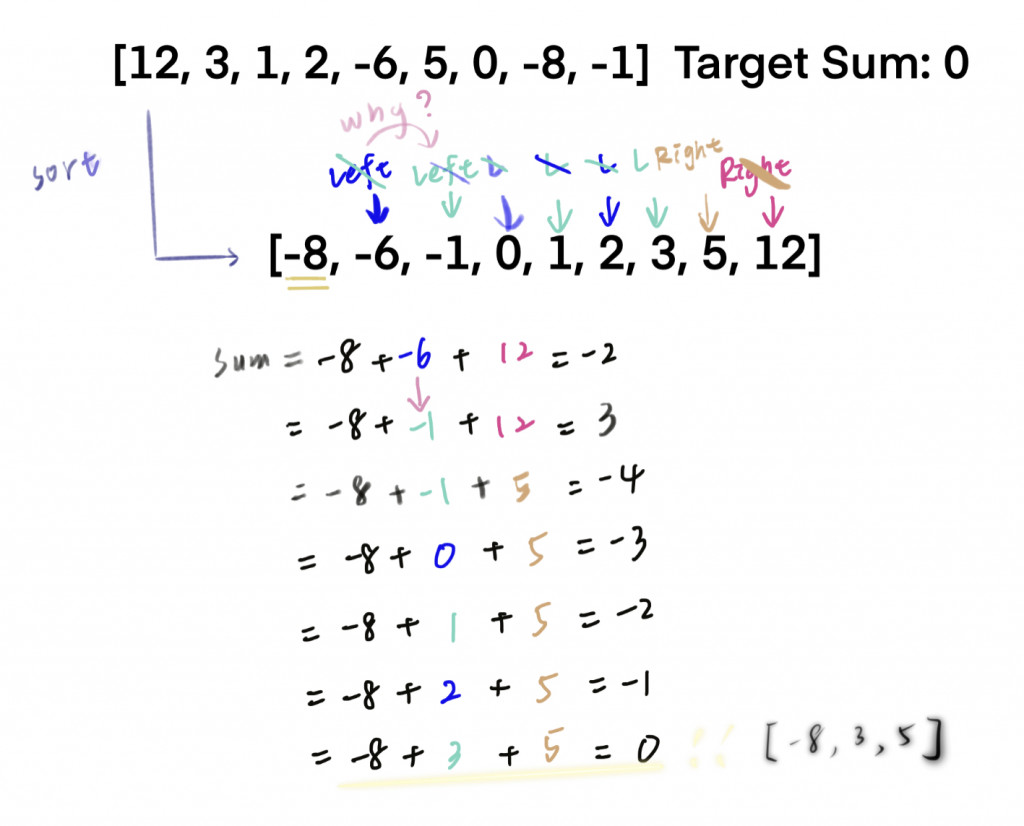#DAY 6
1
Software Development

## Day 06：3 Sum

Target Sum: 0

1.不是空陣列
2.陣列中每數都不同

step1: 我們一樣先將陣列排序
step2: 從陣列的第一個數為主，依序找剩下的數中，可以照到兩個數和第一個數相加，最後達到目標總和``````function threeNumberSum(array, targetSum) {
array.sort((a, b) => a - b);
const triplets = [];
for (let i = 0; i < array.length - 2; i++) {
let left = i + 1;
let right = array.length - 1;
while (left < right) {
const sum = array[i] + array[left] + array[right];
if (sum === targetSum) {
triplets.push([array[i], array[left], array[right]]);
left++;
right--;
} else if (sum < targetSum) {
left++;
} else if (sum > targetSum) {
right--;
}
}
}
return triplets;
}
``````

-> 不可以含重複的triplets

1.重複的基準數要跳過
2.左右指針要移動的時候要先檢查下個數有沒有和自己一樣 -> 如果一樣，就要在往前多走一步

``````var threeSum = function (nums) {
nums.sort((a, b) => a - b);
const triplets = [];

for (let i = 0; i < nums.length - 2; i++) {
if (nums[i] > 0) break;
if (i > 0 && nums[i] === nums[i - 1]) continue;
let left = i + 1;
let right = nums.length - 1;
while (left < right) {
const sum = nums[i] + nums[left] + nums[right];
if (sum === 0) {
triplets.push([nums[i], nums[left], nums[right]]);
while (left < right && nums[left] === nums[left + 1]) left++;
while (left < right && nums[right] === nums[right - 1]) right--;
left++;
right--;
} else if (sum < 0) {
left++;
} else if (sum > 0) {
right--;
}
}
}
return triplets;
};
``````

Squares of a Sorted Array